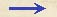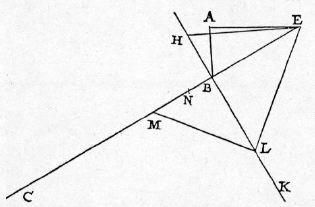Discorsi Propositions 2/35-pr-14 Discorsi Proposition2/35-pr-14{258} PROBLEMA XIV, PROPOSITIO XXXV. {258} PROBLEM XIV, PROPOSITION XXXVData inflexa ad datum perpendiculum, partem in inflexa accipere, in qua sola, ex quiete, fiat motus eodem tempore atque in eadem cum perpendiculo. Given an inclined plane and a limited vertical line, it is required to find a distance on the inclined plane which a body, starting from rest, will traverse in the same time as that needed to traverse both the vertical and the inclined plane. Sit perpendiculum AB, et ad ipsum inflexa BC: oportet, in BC partem accipere, in qua sola, ex quiete, fiat motus eodem tempore ac in eadem cum perpendiculo AB. Ducatur horizon AD, cui inclinata CB extensa occurrat in E, {10} ponaturque BF aequalis BA, et, centro E, intervallo EF, circulus describatur FIG, et FE ad circumferentiam usque protrahatur in G, et ut GB ad BF, ita fiat BH ad HF, et HI circulum tangat in I; deinde ex B perpendicularis ad {20} FC erigatur BK, cui occurrat in L linea EIL; tandem ipsi EL perpendicularis ducatur LM, occurrens BC in M: dico, in linea BM ex quiete in B fieri motum eodem tempore ac ex quiete in A per ambas AB, BM. Ponatur EN aequalis EL; cumque ut GB ad BF, ita sit BH ad HF, erit, permutando, ut GB ad BH, ita BF ad FH, et, dividendo, GH ad HB, ut BH ad HF; quare rectangulum GHF quadrato HB erit aequale: sed idem rectangulum aequatur quoque quadrato HI: ergo BH ipsi HI est aequalis. Cumque in quadrilatero ILBH latera HB, HI sint aequalia, et anguli B, I recti, erit latus quoque BL ipsi LI aequale: {259} est autem EI aequalis EF: ergo tota LE, seu NE, duabus LB, EF est aequalis. Auferatur communis EF; erit reliqua FN ipsi LB aequalis: at posita est FB aequalis ipsi BA: ergo LB duabus AB, BN aequatur. Rursus, si intelligatur, tempus per AB esse ipsam AB, erit tempus per EB ipsi EB aequale; tempus autem per totam EM erit EN, media scilicet inter ME, EB; quare reliquae BM tempus casus post EB, seu post AB, erit ipsa BN: positum autem est, tempus per AB esse AB: ergo tempus casus per ambas ABM est ABN. Cum autem tempus per EB ex quiete in E sit EB, tempus per BM ex quiete in B erit media proportionalis inter BE, BM; haec autem est BL; {10} tempus igitur per ambas ABM ex quiete in A est ABN: tempus vero per BM solam ex quiete in B est BL; ostensum autem est, BL esse aequalem duabus AB, BN; ergo patet propositum. Let AB be the vertical line and BC the inclined plane.It is required to lay off on BC a distance which a body, starting from rest, will traverse in a time equal to that which is occupied by fall through the vertical AB and by descent of the plane. Draw the horizontal line AD, which intersects at E the prolongation of the inclined plane CB; lay off BF equal to BA, and about E as center, with EF as radius describe the circle FIG. Prolong FE until it intersects the circumference at G. Choose a point H such that GB:BF = BH:HF. Draw the line HI tangent to the circle at I. At B draw the line BK perpendicular to FC, cutting the line EIL at L; also draw LM perpendicular to EL and cutting BC at M. Then, I say, BM is the distance which a body, starting from rest at B, will traverse in the same time which is required to descend from rest at A through both distances, AB and BM. Lay off EN equal to EL; then since GB:BF = BH:HF, we shall have, permutando, GB:BH = BF:HF, and, dividendo, GH:BH = BH:HF. Consequently the rectangle GH.HF is equal to the square on BH; but this same rectangle is also equal to the square on HI; therefore BH is equal to HI. Since, in the quadrilateral ILBH, the sides HB and HI are equal and since the angles at B and I are right angles, it follows that the sides BL and LI are also equal: but EI = EF; therefore {259} the total length LE, or NE, is equal to the sum of LB and EF. If we subtract the common part EF, the remainder FN will be equal to LB: but, by construction, FB = BA and, therefore, LB = AB+BN. If again we agree to represent the time of fall through AB by the length AB, (Condition 2/03-th-03-cor) then the time of descent along EB will be measured by EB; (Condition 2/02-th-02-cor2) moreover since EN is a mean proportional between ME and EB it will represent the time of descent along the whole distance EM; (Condition 2/11-th-11) therefore the difference of these distances, BM, will be traversed, after fall from EB, or AB, in a time which is represented by BN. But having already assumed the distance AB as a measure of the time of fall through AB, the time of descent along AB and BM is measured by AB+BN. Since EB measures the time of fall, from rest at E, along EB, (Condition 202C) the time from rest at B along BM will be the mean proportional between BE and BM, namely, BL. The time therefore for the path AB+BM, starting from rest at A is AB+BN; but the time for BM alone, starting from rest at B, is BL; and since it has already been shown that BL = AB+BN, the proposition follows.Aliter, magis expedite. Sit BC planum inclinatum, BA perpendiculum. Ducta perpendiculari per B ad EC, et utrinque extensa, ponatur BH aequalis excessui BE super BA, et angulo BHE ponatur aequalis angulus HEL; ipsa vero EL extensa occurrat BK in L, et ex L {20} excitetur perpendicularis ad EL, LM, occurrens BC in M: dico, BM esse spatium in plano BC quaesitum. Quia enim angulus MLE rectus est, erit BL media inter MB, BE, et LE media inter ME, EB, cui EL secetur aequalis EN; et erunt tres lineae NE, EL, LH aequales, et HB erit excessus NE super BL: verum eadem HB est etiam excessus NE super NB, BA: ergo duae NB, BA aequales sunt BL. Quod si ponatur, EB esse tempus per EB, erit BL {30} tempus per BM ex quiete in B, et BN erit tempus eiusdem post EB, seu post AB, et AB erit tempus per AB: ero tempora per ABM, nempe ABN, aequalia sunt tempori per solam BM ex quiete in B: quod est intentum. Another and shorter proof is the following: Let BC be the inclined plane and BA the vertical; at B draw a perpendicular to EC, extending it both ways; lay off BH equal to the excess of BE over BA; make the angle HEL equal to the angle BHE; prolong EL until it cuts BK in L; at L draw LM perpendicular to EL and extend it till it meets BC in M; then, I say, BM is the portion of BC sought. For, since the angle MLE is a right angle, BL will be a mean proportional between MB and BE, while LE is a mean proportional between ME and BE; lay off EN equal to LE; then NE = EL = LH, and HB = NE-BL. But also HB = NE-(NB+BA); therefore BN+BA = BL. If now we assume the length EB as a measure of the time of descent along EB, (Condition 2/02-th-02-cor2) the time of descent, from rest at B, along BM will be represented by BL; (Condition 2/11-th-11) but, if the descent along BM is from rest at E or at A, then the time of descent will be measured by BN; (Condition 2/03-th-03-cor) and AB will measure the time along AB. Therefore the time required to traverse AB and BM, namely, the sum of the distances AB and BN, is equal to the time of descent, from rest at B, along BM alone. Q. E. F.Discorsi Propositions 2/35-pr-14 Discorsi Proposition2/35-pr-14